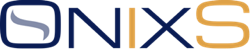# FIXML Tutorial

Version 1.0.0

## Introduction

FIXML is a technical standard for FIX Protocol application-level messages that use XML format. It is widely used for post-trade clearing, settlement, and reporting.

This standard is maintained by FIX Trading Community™ - an independent non-profit, industry-driven standards body.

FIXML is semantically equivalent to the plain text (tag=value) FIX format. In other words, both FIXML and the tag=value FIX formats share the same data dictionary and business rules; the only difference is the representation.

FIXML does not define session layer, FIXML messages can be encapsulated within the FIX Session Protocol using the XML Message <n> message or within other messaging protocols.

## Example

The example below shows a Security Definition <d> message in the FIXML format:

``````
<?xml version="1.0" encoding="UTF-8" ?>

<FIXML v="FIX50SP2" s="20080115">
<SecDef RptID="3400001" ReqID="1234567" RspTyp="100">
<Hdr SID="CME" TID="BRKR" SSub="CPAPI " TSub="user" />
<Instrmt ID="CS" Src="H" ProdCmplx="ENRGY" SecTyp="FUT" MinPxIncr="0.01"
UOM="Bbl" UOMQty="1000" PxUOM="Bbl" PxUOMQty="1" SettlMeth="C"
PxQteMeth="STD" ListMeth="0" TmUnit="Mo" Exch="NYMEX" Desc="
WTI Calendar Swap" />
<InstrmtExt>
<Attrb Typ="29" Val="Y" />
<Attrb Typ="25" Val="1" />
<Attrb Typ="24" Val="2" />
<Attrb Typ="24" Val="12" />
</InstrmtExt>
</SecDef>
</FIXML>
```
```

The equivalent message in the plain text (tag=value) FIX format:

``````
8=FIXT.1.1␁9=271␁35=d␁49=CME␁56=BRKR␁50=CPAPI␁57=user␁1128=9␁320=1234567␁323=100␁48=CS␁22=H␁167=FUT␁207=NYMEX
␁107=WTI Calendar Swap␁969=0.01␁996=Bbl␁997=Mo␁1147=1000␁1227=ENRGY␁1191=Bbl␁1192=1␁1193=C␁1196=STD␁1198=0␁870=4
␁871=29␁872=Y␁871=25␁872=1␁871=24␁872=2␁871=24␁872=12␁964=3400001␁10=246␁
``````

## FIXML Versions

FIXML versions are linked to the versions of the FIX Protocol standard.

## FIXML Schema

The FIXML Schema defines the structure and the legal elements and attributes of a valid FIXML document. It can be used to validate FIXML messages.

FIXML Schemas for FIX Protocol versions FIX 4.1, FIX 4.2, and FIX 4.3 are defined by Document Type Definitions (DTDs), for FIX 4.4 and later – by XML Schemas.

The FIXML Schema can be customized to support business requirements. The FIXML Technical Specification describes the best practices for such customizations.

## FIXML Root Element

FIXML messages are usually placed in the `FIXML` root element that could also include version attributes.

For example:

``````
<?xml version="1.0" encoding="UTF-8" ?>

<FIXML v="FIX50SP2" s="20080115">
<SecDef RptID="3400001" ReqID="1234567" RspTyp="100">
<!--  Message Content here -->
</SecDef>
</FIXML>
```
```

The `"v"` attribute defines the FIX Protocol Version; the `"s"` attribute specifies the FIXML Schema release date.

The FIXML root element can include a single FIXML message or a batch of FIXML messages.

## FIXML Messages

FIX messages are implemented as XML elements.

The FIXML element name is the abbreviated name of the message. For example:

## FIXML Fields

FIX message fields are implemented as the message element attributes.

The attribute name is the abbreviated name of the FIX message field. For example:

``````
<SecDef RptID="3400001" ReqID="1234567" RspTyp="100">
``````

To find the tag number of the field, use the description of its type. For example:

``````
<xs:attributeGroup name="SecurityDefinitionAttributes">
<xs:attribute name="RptID" type="SecurityReportID_t" use="optional"/>
``````

And

``````
<xs:simpleType name="SecurityReportID_t">
<xs:annotation>
<xs:documentation>Identifies a Security List message.</xs:documentation>
<xs:appinfo>
<fm:Xref Protocol="FIX" name="SecurityReportID" ComponentType="Field" Tag="964" Type="int" AbbrName="RptID"/>
</xs:appinfo>
</xs:annotation>
<xs:restriction base="xs:integer"/>
</xs:simpleType>
``````

## FIXML Components

The FIXML Schema defines FIXML Components - reusable sequences of related fields grouped inside the component element. The same components appear in multiple messages. For example:

``````
<InstrmtExt>
<Attrb Typ="29" Val="Y"/>
<Attrb Typ="25" Val="1"/>
<Attrb Typ="24" Val="2"/>
<Attrb Typ="24" Val="12"/>
</InstrmtExt>
``````

## FIXML Datatypes

The FIXML Schema contains definitions for the FIXML datatypes. Many of them are based upon ISO datatypes, so some of the FIXML datatypes differ from FIX tag=value datatypes. For example, the FIX representation of the UTCTimestamp is different from the FIXML representation, so the TransactTime <60> field in FIXML is

``````
TxnTm="2001-09-11T09:30:47-05:00"
``````

But in the plain text (tag=value) FIX, it is

``````
60=20010911-09:30:47-05:00
``````

The conversion between FIX tag=value datatypes and FIXML is not standardized and left to implementers of FIX <-> FIXML Converters.

## FIXML Parsers

FIXML uses XML syntax, so an XML Parser can parse it.

## FIX <-> FIXML Converters

FIX Engine implementations include the ability to convert from FIXML to FIX format and vice versa. For example:

## FIXML versus FIX

Although FIXML is logically equivalent to the tag=value FIX format, the difference in representation has the following advantages and disadvantages:

FIXML
• Easy interoperability